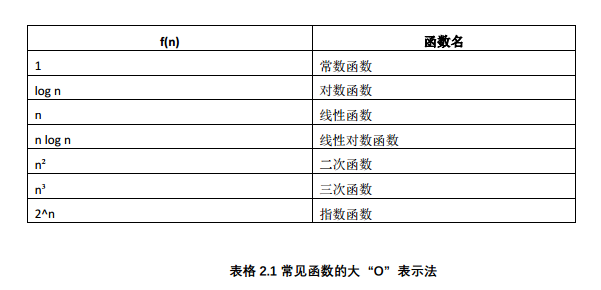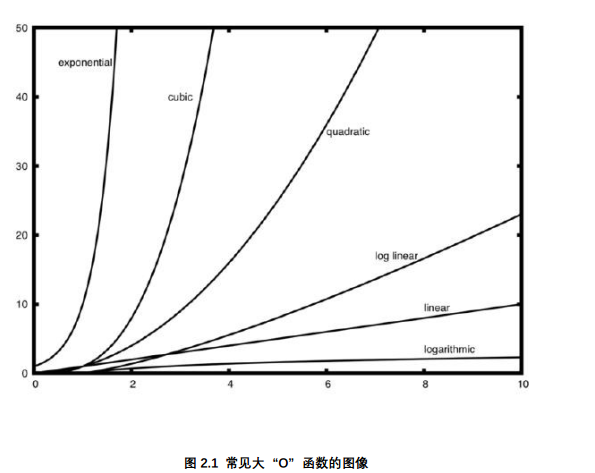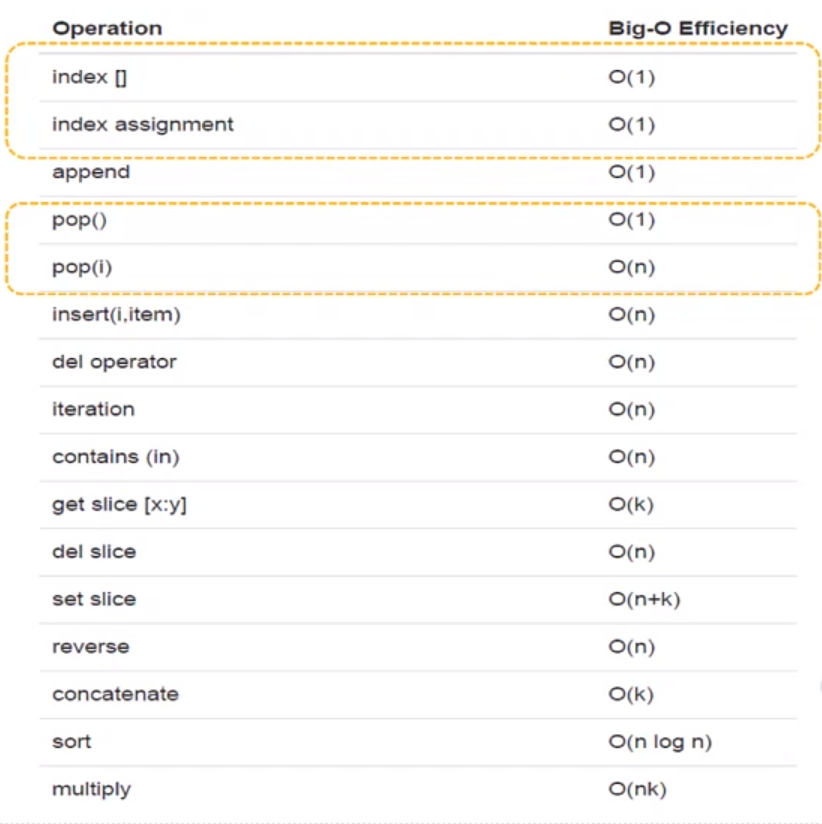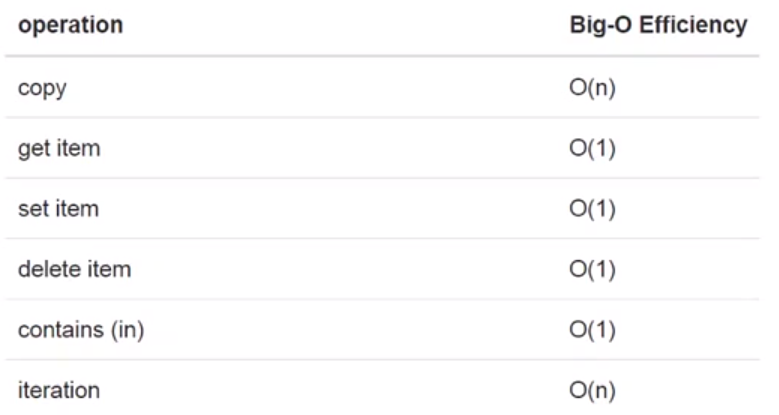## 什么是算法分析

• 算法是问题解决的通用的分步的指令的聚合
• 算法分析主要就是从计算资源的消耗的角度来评判和比较算法。

• 存储空间或内存
• 执行时间

## 影响算法运行时间的其他因素

• 分为最好、最差和平均情况，平均状况体现主流性能

## 累计求和案例

``````import time

def sumOFN2(n):
start=time.time()
theSum=0
for i in range(1,n+1):
theSum+=i
end=time.time()
return theSum,end-start

def sumOFN3(n):
start=time.time()
theSum=(n*(n+1))/2
end=time.time()
return theSum,end-start

if __name__ == "__main__":
for i in range(5):
print("sum is %d required %10.7f seconds" % sumOFN2(100000))
print("--------")
for i in range(5):
print("sum is %d required %10.7f seconds" % sumOFN3(100000))

-----------
sum is 5000050000 required  0.1255534 seconds
sum is 5000050000 required  0.1245010 seconds
sum is 5000050000 required  0.1389964 seconds
sum is 5000050000 required  0.1279476 seconds
sum is 5000050000 required  0.1276410 seconds
--------
sum is 5000050000 required  0.0000000 seconds
sum is 5000050000 required  0.0000000 seconds
sum is 5000050000 required  0.0000000 seconds
sum is 5000050000 required  0.0000000 seconds
sum is 5000050000 required  0.0000000 seconds
``````

## “大O” 表示法

• 数量级函数用来描述当规模 n 增加时， T(n)函数中增长最快的部分。

• 常见大O表示法## 变 位 词 检 测

• 逐字检查法 O(n²)
• 排序比较法 O(nlogn)
• 暴力匹配算法 O(n!)
• 字母计数比较法 O(n)
``````#变位词判断问题
#逐字检查
def anagramSolution(s1,s2):
alist=list(s2)
pos1=0
stillOK=True
while pos1<len(s1) and stillOK:#循环s1中的每一个字符
pos2=0
found=False
if s1[pos1]==alist[pos2]:
found=True
else:
pos2=pos2+1
if found:
alist[pos2]=None
else:
stillOK=False
pos1=pos1+1
return stillOK

#print(anagramSolution('abcd','dcba'))

#排序解法
def anagramSolution2(s1,s2):
alist1=list(s1)
alist2=list(s2)
alist1.sort()
alist2.sort()
pos=0
matches=True
while pos<len(s1) and matches:
if alist1[pos]==alist2[pos]:
pos=pos+1
else:
matches=False
return matches
print(anagramSolution2('abcd','dcba'))

#计数器解法
def anagramSolution3(s1,s2):
c1=*26
c2=*26
for i in range(len(s1)):
pos=ord(s1[i])-ord('a')#把a-z转化为0-25   ps:感谢m0_47550366的提醒，此处错误已改正
c1[pos]=c1[pos]+1#计数
for i in range(len(s2)):
pos = ord(s2[i]) - ord('a')  # 把a-z转化为0-25
c2[pos] = c2[pos] + 1  # 计数
j=0
stillOK=True
while j<26 and stillOK:
if c1[j]==c2[j]:
j=j+1
else:
stillOK=False
return stillOK
print(anagramSolution3('abcd','dcba'))

``````

## python数据类型

### List 列表

`````````python
import timeit # 运行时间测量模块
from timeit import Timer
# 循环连接
def test1():
l=[]
for i in range(1000):
l=l+[i]

# append添加
def test2():
l=[]
for i in range(1000):
l.append(i)

# 列表推导式
def test3():
l=[i for i in range(1000)]

# range转列表
def test4():
l=list(range(1000))

if __name__ == "__main__":
t1=Timer("test1()","from __main__ import test1")
print("concat %f second" % t1.timeit(number=1000)) # number指定程序被执行的次数

t2=Timer("test2()","from __main__ import test2")
print("append %f second" % t2.timeit(number=1000))

t3=Timer("test3()","from __main__ import test3")
print("comprehension %f second" % t3.timeit(number=1000))

t4=Timer("test4()","from __main__ import test4")
print("list range %f second" % t4.timeit(number=1000))

-------
concat 1.979591 second
append 0.811599 second
comprehension 0.375291 second
list range 0.024658 second
```
``````### dict 字典## 【数据结构与算法Python版学习笔记】算法分析的更多相关文章

1. 【数据结构与算法Python版学习笔记】引言

学习来源 北京大学-数据结构与算法Python版 目标 了解计算机科学.程序设计和问题解决的基本概念 计算机科学是对问题本身.问题的解决.以及问题求解过程中得出的解决方案的研究.面对一 个特定问题,计 ...

2. 【数据结构与算法Python版学习笔记】查找与排序——散列、散列函数、区块链

散列 Hasing 前言 如果数据项之间是按照大小排好序的话,就可以利用二分查找来降低算法复杂度. 现在我们进一步来构造一个新的数据结构, 能使得查找算法的复杂度降到O(1), 这种概念称为" ...

3. 【数据结构与算法Python版学习笔记】基本数据结构——列表 List，链表实现

无序表链表 定义 一种数据项按照相对位置存放的数据集 抽象数据类型无序列表 UnorderedList 方法 list() 创建一个新的空列表.它不需要参数,而返回一个空列表. add(item) 将 ...

4. 【数据结构与算法Python版学习笔记】目录索引

引言 算法分析 基本数据结构 概览 栈 stack 队列 Queue 双端队列 Deque 列表 List,链表实现 递归(Recursion) 定义及应用:分形树.谢尔宾斯基三角.汉诺塔.迷宫 优化 ...

5. 【数据结构与算法Python版学习笔记】递归(Recursion)——定义及应用：分形树、谢尔宾斯基三角、汉诺塔、迷宫

定义 递归是一种解决问题的方法,它把一个问题分解为越来越小的子问题,直到问题的规模小到可以被很简单直接解决. 通常为了达到分解问题的效果,递归过程中要引入一个调用自身的函数. 举例 数列求和 def ...

6. 【数据结构与算法Python版学习笔记】图——最短路径问题、最小生成树

最短路径问题 概念 可以通过"traceroute"命令来跟踪信息传送的路径: traceroute www.lib.pku.edu.cn 可以将互联网路由器体系表示为一个带权边的 ...

7. 【数据结构与算法Python版学习笔记】图——骑士周游问题 深度优先搜索

骑士周游问题 概念 在一个国际象棋棋盘上, 一个棋子"马"(骑士) , 按照"马走日"的规则, 从一个格子出发, 要走遍所有棋盘格恰好一次.把一个这样的走棋序列 ...

8. 【数据结构与算法Python版学习笔记】图——词梯问题 广度优先搜索 BFS

词梯Word Ladder问题 要求是相邻两个单词之间差异只能是1个字母,如FOOL变SAGE: FOOL >> POOL >> POLL >> POLE > ...

9. 【数据结构与算法Python版学习笔记】树——利用二叉堆实现优先级队列

概念 队列有一个重要的变体,叫作优先级队列. 和队列一样,优先级队列从头部移除元素,不过元素的逻辑顺序是由优先级决定的. 优先级最高的元素在最前,优先级最低的元素在最后. 实现优先级队列的经典方法是使 ...

## 随机推荐

1. 第20篇-加载与存储指令之ldc与_fast_aldc指令（2）

ldc指令将int.float.或者一个类.方法类型或方法句柄的符号引用.还可能是String型常量值从常量池中推送至栈顶. 这一篇介绍一个虚拟机规范中定义的一个字节码指令ldc,另外还有一个虚拟机内 ...

2. IPSEC的实现方式

IPSEC的实现方式 在IPSEC通信中涉及到一个重要方面,那就是如何定义要保护的数据流(又称为感兴趣流).这不仅涉及到IPSEC最终要保护哪部分数据,还关系到IPSEC的实现方式,因此有必要把感兴趣 ...

3. [推荐]MyBatis 核心技术与面试 34 讲

MyBatis 核心技术与面试 34 讲 职业生涯中常被问到: 如何成为某方面的高手? 如何快速搞定某项技术? 我现在的水平处于什么阶段? -- 我暗暗想,我们从小学到中学到大学,经历了大考三六九.小 ...

4. 无序数组求第K大的数

问题描述 无序数组求第K大的数,其中K从1开始算. 例如:[0,3,1,8,5,2]这个数组,第2大的数是5 OJ可参考:LeetCode_0215_KthLargestElementInAnArra ...

5. 快乐中秋，SQL小白入门指南

目录 创建表 最基本的创建 怎么查看一个已经建好的表的信息呢 修改字段 插入数据 修改和删除数据 修改 删除 第一个查询 条件语句 使用age的大小比较,查看大于16岁的学生: 使用多个条件并联,大于 ...

6. Docker系列（4）- run的流程和docker原理

回顾HelloWorld流程 底层工作原理 Docker是怎么工作的? Docker是一个Client-Server结构的系统,Docker的守护进程运行在宿主机上.通过Socket从客户端访问 Do ...

7. JDBC-3(Transcation) ****

3.1 异常的使用说明 在工具类中(JDBCUtils)的方法最好声明异常(throws),以便后续实现类中去捕获这些异常. 工具类中捕获异常通常没有意义 eg:实现类中connection建立过程出 ...

8. 写SQL的套路

定义问题 转化问题 如要解决的问题是:查出每门课程成绩都大于80分学生的姓名,可以转化为:只要学生最小分数的课程大于80分,就是所有课程成绩都大于80分. 查询同名同姓学生名单并统计同名人数--> ...

9. 虚拟机安装配置centos7

安装 https://blog.csdn.net/babyxue/article/details/80970526 主机环境预设 更换国内yum源 epel源 https://www.cnblogs. ...

10. MyBatis Plus 批量数据插入功能，yyds！

最近 Review 小伙伴代码的时候,发现了一个小小的问题,小伙伴竟然在 for 循环中进行了 insert (插入)数据库的操作,这就会导致每次循环时都会进行连接.插入.断开连接的操作,从而导致一定 ...